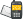It is currently 26 May 2019, 08:11### GMAT Club Daily Prep

#### Thank you for using the timer - this advanced tool can estimate your performance and suggest more practice questions. We have subscribed you to Daily Prep Questions via email.

Customized
for You

we will pick new questions that match your level based on your Timer History

Track
Your Progress

every week, we’ll send you an estimated GMAT score based on your performance

Practice
Pays

we will pick new questions that match your level based on your Timer History

#### Not interested in getting valuable practice questions and articles delivered to your email? No problem, unsubscribe here.# If mn < 0 and k/m + l/n < mnQuestion banks Downloads My Bookmarks Reviews Important topics
Author Message
TAGS:
FounderJoined: 18 Apr 2015
Posts: 6643
Followers: 107

Kudos [?]: 1274 , given: 6003

If mn < 0 and k/m + l/n < mn [#permalink]
Expert's post00:00

Question Stats:60% (00:56) correct39% (01:01) wrongbased on 33 sessions
If $$mn < 0$$ and $$\frac{k}{m} + \frac{l}{n} < mn$$, which of the following must be true ?

(A) $$km + ln < (mn)^2$$

(B) $$kn + lm < 1$$

(C) $$kn + lm > (mn)^2$$

(D) $$k + l > mn$$

(E) $$km > –ln$$
[Reveal] Spoiler: OA

_________________
DirectorJoined: 09 Nov 2018
Posts: 509
Followers: 0

Kudos [?]: 23 , given: 1

Re: If mn < 0 and k/m + l/n < mn [#permalink]
Explain please.GRE InstructorJoined: 10 Apr 2015
Posts: 1800
Followers: 58

Kudos [?]: 1681  , given: 8

Re: If mn < 0 and k/m + l/n < mn [#permalink]
2
This post received
KUDOS
Expert's post
Carcass wrote:
If $$mn < 0$$ and $$\frac{k}{m} + \frac{l}{n} < mn$$, which of the following must be true ?

(A) $$km + ln < (mn)^2$$

(B) $$kn + lm < 1$$

(C) $$kn + lm > (mn)^2$$

(D) $$k + l > mn$$

(E) $$km > –ln$$

GIVEN: $$\frac{k}{m} + \frac{l}{n} < mn$$
Rewrite fractions with common denominator: $$\frac{kn}{mn} + \frac{lm}{mn} < mn$$
Combine fractions: $$\frac{kn+lm}{mn} < mn$$
Multiply both sides of inequality by $$mn$$ to get: $$kn+lm > (mn)^2$$

ASIDE: Since we're told that $$mn < 0$$, we REVERSED the direction of the inequality symbol, because in the last step, we multiplied both sides by a NEGATIVE value.

Answer: C

Cheers,
Brent
_________________

Brent Hanneson – Creator of greenlighttestprep.comSign up for my free GRE Question of the Day emailsInternJoined: 07 Aug 2016
Posts: 42
Followers: 0

Kudos [?]: 15  , given: 0

Re: If mn < 0 and k/m + l/n < mn [#permalink]
1
This post received
KUDOS
AE wrote:
Explain please.

k/m + l/n < mn

It becomes (kn + lm)/mn < mn

We multiplty by mn. Since it is is a negative number it will reverse the inequality sign.

Becoming kn + lm > (mn)^2

Posted from my mobile deviceManagerJoined: 23 Oct 2018
Posts: 57
Followers: 0

Kudos [?]: 0 , given: 0

Re: If mn < 0 and k/m + l/n < mn [#permalink]
thanksRe: If mn < 0 and k/m + l/n < mn   [#permalink] 27 Nov 2018, 21:38
Display posts from previous: Sort by

# If mn < 0 and k/m + l/n < mnQuestion banks Downloads My Bookmarks Reviews Important topicsPowered by phpBB © phpBB Group Kindly note that the GRE® test is a registered trademark of the Educational Testing Service®, and this site has neither been reviewed nor endorsed by ETS®.